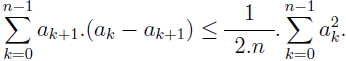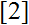# Volume 18, 2012, Number 3

Volume 18Number 1Number 2 ▷ Number 3 ▷ Number 4

In Memoriam: Prof. Antal Bege (1962–2012)
Editorial. Pages 1—4
J. Sándor
Editorial (PDF, 107 Kb)

A modification of an elementary numerical inequality
Original research paper. Pages 5—7
Krassimir Atanassov
Full paper (PDF, 109 Kb) | Abstract

It is proved that for every real numbers a0, a1, …, an (n ≥ 1):This is a modification of an inequality, previously introduced by the author.

Odd-powered triples and Pellian sequences
Original research paper. Pages 8—12
J. V. Leyendekkers and A. G. Shannon
Full paper (PDF, 123 Kb) | Abstract

Even and odd integers of the form Nm in the modular ring Z4 have rows with elements which satisfy Pellian recurrence relations from which Pythagorean triples can form when m = 2. When m is odd, they have different and incompatible Pellian row structure and triples are not formed.

Fibonacci numbers at most one away from a product of factorials
Original research paper. Pages 13—19
Diego Marques
Full paper (PDF, 166 Kb) | Abstract

Let (Fn)n≥0 be the Fibonacci sequence given by F0 = 0; F1 = 1 and Fn+2 = Fn+1 + Fn, for n ≥ 0. In this note, we find all solutions of the Diophantine equation m1! … mk! ± 1 = Fm, where 2 ≤ m1 ≤ … ≤ mk and m ≥ 3.

Isomorphism testings for graph CG(a, b)
Original research paper. Pages 20—34
Full paper (PDF, 234 Kb) | Abstract

Let G be a finite abelian group such that G ≠ {0} and a, bG \ {0}. Let CG(a, b) be the graph whose vertex set is G and the edge set is given by
E = {{x, x + a}, {x, x + b}, {x, x − a}, {x, x − b} : xG}.
In this work, we use the properties of finite abelian group to derive isomorphism testing on the graph CG(a, b) defined above. We study classes of isomorphic graphs. This work generalizes Nicoloso and Pietropaoli’s paper.

A new elementary proof of the inequality φ(n) > π (n)
Original research paper. Pages 35—37
Carlo Sanna
Full paper (PDF, 131 Kb) | Abstract

In this paper we provide a new elementary proof that the inequality φ(n) > π(n) holds for all integers n ≥ 91, an old result of L. Moser. Our proof is based on Bonse’s Inequality. This makes it somewhat simpler than Moser’s proof, which in turn relies on Bertrand’s Postulate.

Generalized differential operators
Original research paper. Pages 38—44
A. G. Shannon
Full paper (PDF, 44 Kb) | Abstract

This paper considers some properties of generalized differential operators by extending Chak and Schur derivatives as previously investigated by Leonard Carlitz. They are applied in the context of extended Laguerre polynomials.

A note on sumsets and difference sets in ℤ/nℤ
Original research paper. Pages 45—47
Christopher J. Richardson and Craig V. Spencer
Full paper (PDF, 168 Kb) | Abstract

In this brief note, we investigate the quantity k(n), which is the smallest natural number r such that for all subsets A  ℤ/nℤ satisfying A ⊆ A = ℤ/nℤ, we have rA = ℤ/nℤ.

The Irrationals: A Story of the Numbers You Can’t Count On by Julian Havil
Book review. Page 48
Anthony Shannon
Book review (PDF, 107 Kb)

Volume 18Number 1Number 2 ▷ Number 3 ▷ Number 4